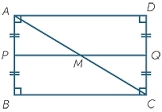# In the

In the rectangle ABCD, the distance of its center from the line AB is 3 cm greater than from the line BC. The circumference of the rectangle is 52 cm. Calculate the contents of the rectangle. Express the result in cm2.

Correct result:

S =  160 cm2

#### Solution:

$o=52 \ \text{cm} \ \\ \ \\ \ \\ o=2 \cdot \ (a+b) \ \\ b/2=3 + a/2 \ \\ 52=2 \cdot \ (a+b) \ \\ b/2=3 + a/2 \ \\ \ \\ 2a+2b=52 \ \\ a-b=-6 \ \\ \ \\ a=10 \ \\ b=16 \ \\ \ \\ \ \\ S=a \cdot \ b=10 \cdot \ 16=160 \ \text{cm}^2$Our examples were largely sent or created by pupils and students themselves. Therefore, we would be pleased if you could send us any errors you found, spelling mistakes, or rephasing the example. Thank you!

Please write to us with your comment on the math problem or ask something. Thank you for helping each other - students, teachers, parents, and problem authors.Tips to related online calculators
Do you have a system of equations and looking for calculator system of linear equations?

#### You need to know the following knowledge to solve this word math problem:

We encourage you to watch this tutorial video on this math problem:

## Next similar math problems:

• Ratio of sidesCalculate the area of a circle that has the same circumference as the circumference of the rectangle inscribed with a circle with a radius of r 9 cm so that its sides are in ratio 2 to 7.
• BillboardRectangular billboard is 2.5 m long with a diagonal 2.8 m long. Calculate the perimeter and the content area of the billboard.Show that the quadrilateral with vertices P1(0,1), P2(4,2) P3(3,6) P4(-5,4) has two right triangles.
• Circular poolThe base of the pool is a circle with a radius r = 10 m, excluding a circular segment that determines the chord length 10 meters. The pool depth is h = 2m. How many hectoliters of water can fit into the pool?
• Area of a rectangleCalculate the area of a rectangle with a diagonal of u = 12.5cm and a width of b = 3.5cm. Use the Pythagorean theorem.
• DecagonCalculate the area and circumference of the regular decagon when its radius of a circle circumscribing is R = 1m
• 30-gonAt a regular 30-gon the radius of the inscribed circle is 15cm. Find the "a" side size, circle radius "R", circumference, and content area.
• Quarter circleWhat is the radius of a circle inscribed in the quarter circle with a radius of 100 cm?
• WasteHow many percents are waste from a circular plate with a radius of 1 m from which we cut a square with the highest area?
• NonagonCalculate the area and perimeter of a regular nonagon if its radius of inscribed circle is r = 10cm
• Circular ringSquare with area 16 centimeters square are inscribed circle k1 and described circle k2. Calculate the area of circular ring, which circles k1, k2 form.
• FlakesA circle was described on the square, and a semicircle above each side of the square was described. This created 4 "flakes". Which is bigger: the content of the central square or the content of four chips?
• Annular areaThe square with side a = 1 is inscribed and circumscribed by circles. Find the annular area.
• Circular segmentCalculate the area S of the circular segment and the length of the circular arc l. The height of the circular segment is 2 cm and the angle α = 60°. Help formula: S = 1/2 r2. (Β-sinβ)
• The trapeziumThe trapezium is formed by cutting the top of the right-angled isosceles triangle. The base of the trapezium is 10 cm and the top is 5 cm. Find the area of trapezium.
• Company logoThe company logo consists of a blue circle with a radius of 4 cm, which is an inscribed white square. What is the area of the blue part of the logo?
• SquareCalculate the perimeter and the area of square with a diagonal length 30 cm.